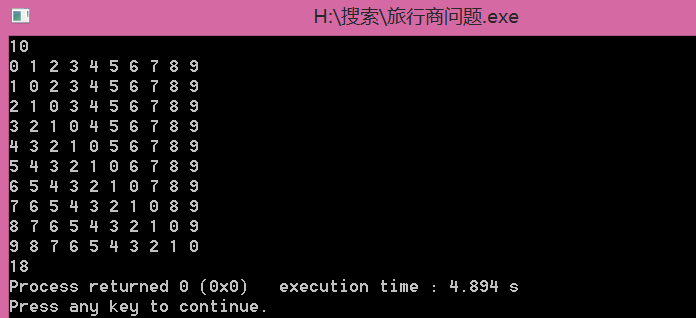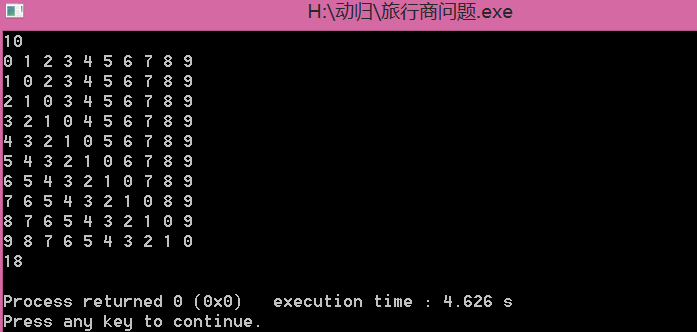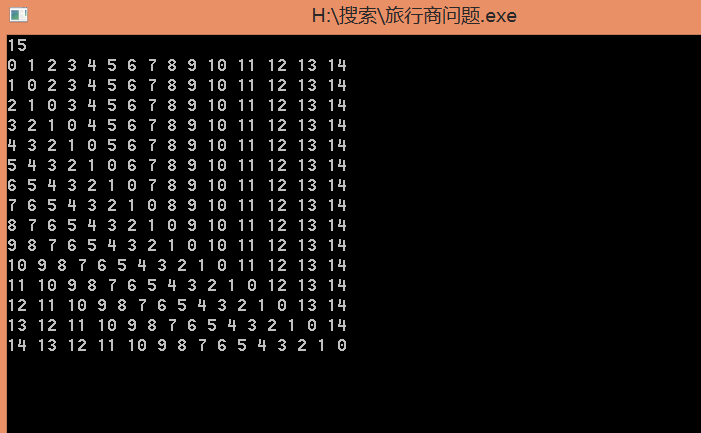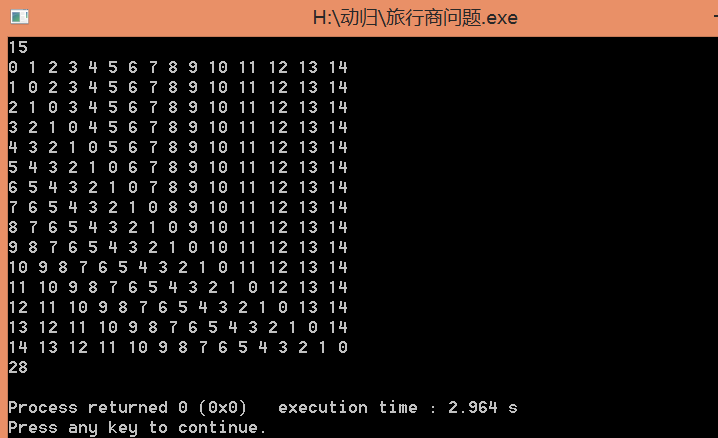# 旅行商问题（状态压缩的DP）

#include<iostream>
#include<cstdio>
#include<algorithm>
using namespace std;
int a;
int ans=0x3f3f3f3f;
int n;
int flag={0};
void dfs(int start,int num,int sum)
{
if(num==n)
{
ans=min(ans,sum+a[start]);
return ;
}
for(int i=2;i<=n;i++)
{
if(flag[i]==0)
{
flag[i]=1;
dfs(i,num+1,sum+a[start][i]);
flag[i]=0;
}
}
}

int main()
{
scanf("%d",&n);
for(int i=1;i<=n;i++)
for(int j=1;j<=n;j++)
scanf("%d",&a[i][j]);
flag=1;
dfs(1,1,0);
printf("%d",ans);
return 0;
}



1&1=1
1&0=0

1|1=1
1|0=1
0|0=0

K位置上值清零，其余位不变   s&(~1<<(k-1))

K位置上值变为1，其余位不变  s|(1<<(k-1))

1的二进制位为：00000001，如果它要移动到k位置它只需要左移k-1位置就可以，左移后除了k位置上的值为1，其余位全为0，所以相或的那个数的k位置上的0会变为1，其余位置上的值不发生改变；同样道理把1左移k-1后，把它全部按位取反，，则除了1左移后k位置上的值为0，其余全为1,因为1&1=1，1&0=0，所以相与的那个数的其他位置值都不发生改变，而0&1=0，0&0=0，所以这样可以使原来k位置上的1变为0.所以上面那两个公式的正确性可以保证~~

24:00011000   1:00000001
1左移三，则1（左移）：00001000 按位取反：11110111

把24的第3个位置上的0变为1：24|(1<<(3-1))
1左移二，则1（左移）：00000100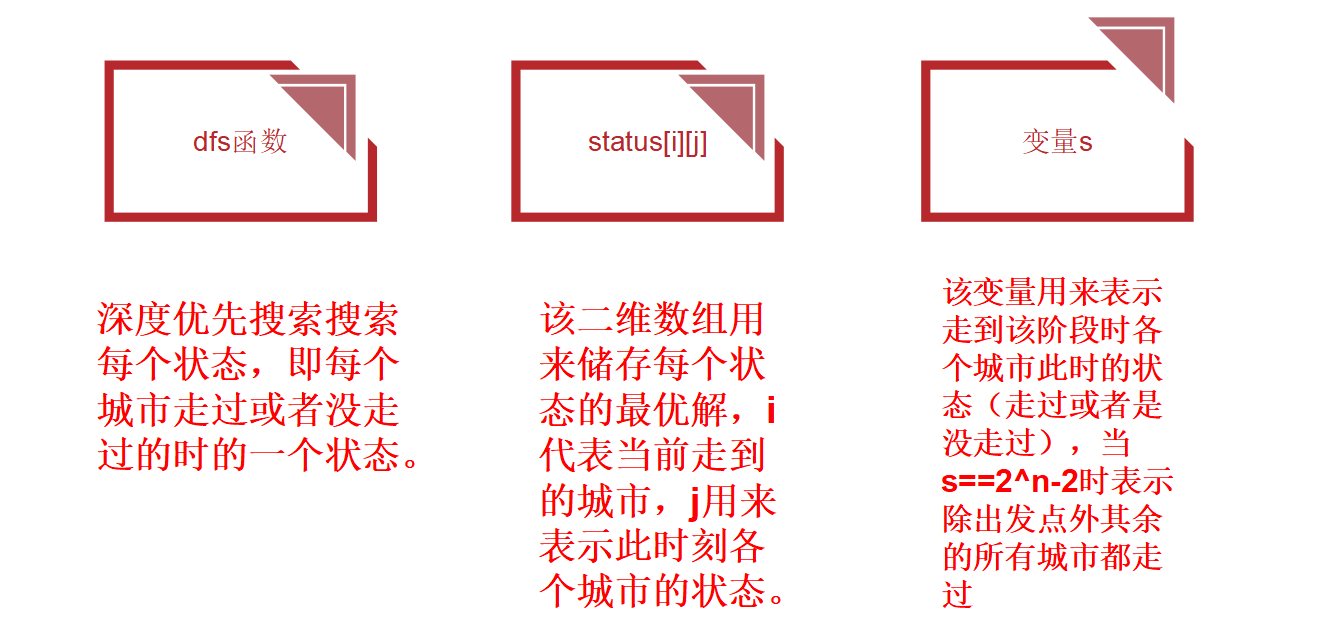#include<bits/stdc++.h>
using namespace std;
int DFS(int v,int S);
int n,Map,maxi;
int status;
int main()
{
scanf("%d",&n);
maxi=(int)pow(2.0,n*1.0);
for(int i=1;i<=n;i++)
for(int j=1;j<=n;j++)
scanf("%d",&Map[i][j]);
cout<<DFS(1,0)<<endl;
return 0;
}
int DFS(int v,int S){
if(S==(maxi-2)) return  Map[v];//发现所有的城市都走过后结束
if(status[v][S]) return status[v][S];//如果当前状态已经求解过直接
int M=99999999;                              //返回
for(int i=2;i<=n;i++)//查找没有走过的城市
if(!(S&(1<<(i-1)))&&v!=i){
S|=(1<<(i-1));                                  //要走该城市则在对应位置变值
M=min(M,DFS(i,S)+Map[v][i]);      //为1表示走过
S&=(~(1<<(i-1)));                             //把刚才走过的城市变回为0，
}                                                        //给其他情况使用，方便找最优
return status[v][S]=M;
}